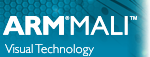OpenGL ES SDK for Android ARM Developer Center
FFTWater Class Reference

`#include <fftwater.hpp>`

## Public Member Functions

FFTWater (float amplitude, vec2 wind_velocity, uvec2 resolution, vec2 size, vec2 normalmap_freq_mod)

void update (float time)

GLuint get_height_displacement () const

GLuint get_gradient_jacobian () const

GLuint get_normal () const

unsigned get_displacement_downsample () const

## Private Member Functions

cfloat phillips (vec2 k, float max_l)

void generate_distribution (cfloat *distribution, vec2 size, float amplitude, float max_l)

void generate_mipmaps ()

void compute_ifft ()

void update_phase (float time)

void init_gl_fft ()

void downsample_distribution (cfloat *out, const cfloat *in, unsigned rate_log2)

void compute_mipmap (const GLFFT::Program &program, const GLFFT::Texture &texture, GLenum format, unsigned Nx, unsigned Nz, unsigned level)

void init_texture (GLFFT::Texture &tex, GLenum format, unsigned levels, unsigned width, unsigned height, GLenum mag_filter, GLenum min_filter)

## Private Attributes

vec2 wind_velocity

vec2 wind_dir

unsigned Nx

unsigned Nz

vec2 size

vec2 size_normal

float L

std::normal_distribution< floatnormal_dist {0.0f, 1.0f}

std::default_random_engine engine

std::vector< cfloatdistribution

std::vector< cfloatdistribution_displacement

std::vector< cfloatdistribution_normal

GLFFT::Program prog_generate_height

GLFFT::Program prog_generate_normal

GLFFT::Program prog_generate_displacement

GLFFT::Program prog_mipmap_height

GLFFT::Program prog_mipmap_normal

GLFFT::Texture heightmap 

GLFFT::Texture displacementmap 

GLFFT::Texture normalmap 

GLFFT::Texture heightdisplacementmap 

unsigned texture_index = 0

unsigned normal_levels = 0

unsigned displacement_downsample = 0

GLFFT::Buffer distribution_buffer

GLFFT::Buffer distribution_buffer_displacement

GLFFT::Buffer distribution_buffer_normal

GLFFT::Buffer freq_height

GLFFT::Buffer freq_displacement

GLFFT::Buffer freq_normal

std::unique_ptr< GLFFT::FFTfft_height

std::unique_ptr< GLFFT::FFTfft_displacement

std::unique_ptr< GLFFT::FFTfft_normal

bool mipmap_fp16

## Static Private Attributes

static constexpr float G = 9.81f

## Detailed Description

Definition at line 34 of file fftwater.hpp.

## Constructor & Destructor Documentation

 FFTWater::FFTWater ( float amplitude, vec2 wind_velocity, uvec2 resolution, vec2 size, vec2 normalmap_freq_mod )

Definition at line 33 of file fftwater.cpp.

## Member Function Documentation

 void FFTWater::bake_height_gradient ( )
private

Definition at line 235 of file fftwater.cpp.

 void FFTWater::compute_ifft ( )
private

Definition at line 176 of file fftwater.cpp.

 void FFTWater::compute_mipmap ( const GLFFT::Program & program, const GLFFT::Texture & texture, GLenum format, unsigned Nx, unsigned Nz, unsigned level )
private

Definition at line 263 of file fftwater.cpp.

 void FFTWater::downsample_distribution ( cfloat * out, const cfloat * in, unsigned rate_log2 )
private

Definition at line 76 of file fftwater.cpp.

 void FFTWater::generate_distribution ( cfloat * distribution, vec2 size, float amplitude, float max_l )
private

Definition at line 124 of file fftwater.cpp.

 void FFTWater::generate_mipmaps ( )
private

Definition at line 187 of file fftwater.cpp.

 unsigned FFTWater::get_displacement_downsample ( ) const
inline

Definition at line 109 of file fftwater.hpp.

 GLuint FFTWater::get_gradient_jacobian ( ) const
inline

Definition at line 107 of file fftwater.hpp.

 GLuint FFTWater::get_height_displacement ( ) const
inline

Definition at line 106 of file fftwater.hpp.

 GLuint FFTWater::get_normal ( ) const
inline

Definition at line 108 of file fftwater.hpp.

 void FFTWater::init_gl_fft ( )
private

Definition at line 294 of file fftwater.cpp.

 void FFTWater::init_texture ( GLFFT::Texture & tex, GLenum format, unsigned levels, unsigned width, unsigned height, GLenum mag_filter, GLenum min_filter )
private

Definition at line 288 of file fftwater.cpp.

 cfloat FFTWater::phillips ( vec2 k, float max_l )
private

Definition at line 104 of file fftwater.cpp.

 void FFTWater::update ( float time )

Definition at line 226 of file fftwater.cpp.

 void FFTWater::update_phase ( float time )
private

Definition at line 143 of file fftwater.cpp.

## Member Data Documentation

 unsigned FFTWater::displacement_downsample = 0
private

Definition at line 78 of file fftwater.hpp.

 GLFFT::Texture FFTWater::displacementmap
private

Definition at line 70 of file fftwater.hpp.

 std::vector FFTWater::distribution
private

Definition at line 56 of file fftwater.hpp.

 GLFFT::Buffer FFTWater::distribution_buffer
private

Definition at line 80 of file fftwater.hpp.

 GLFFT::Buffer FFTWater::distribution_buffer_displacement
private

Definition at line 81 of file fftwater.hpp.

 GLFFT::Buffer FFTWater::distribution_buffer_normal
private

Definition at line 82 of file fftwater.hpp.

 std::vector FFTWater::distribution_displacement
private

Definition at line 57 of file fftwater.hpp.

 std::vector FFTWater::distribution_normal
private

Definition at line 58 of file fftwater.hpp.

 std::default_random_engine FFTWater::engine
private

Definition at line 53 of file fftwater.hpp.

 std::unique_ptr FFTWater::fft_displacement
private

Definition at line 87 of file fftwater.hpp.

 std::unique_ptr FFTWater::fft_height
private

Definition at line 86 of file fftwater.hpp.

 std::unique_ptr FFTWater::fft_normal
private

Definition at line 88 of file fftwater.hpp.

 GLFFT::Buffer FFTWater::freq_displacement
private

Definition at line 84 of file fftwater.hpp.

 GLFFT::Buffer FFTWater::freq_height
private

Definition at line 83 of file fftwater.hpp.

 GLFFT::Buffer FFTWater::freq_normal
private

Definition at line 85 of file fftwater.hpp.

 constexpr float FFTWater::G = 9.81f
staticprivate

Definition at line 54 of file fftwater.hpp.

private

Definition at line 74 of file fftwater.hpp.

 GLFFT::Texture FFTWater::heightdisplacementmap
private

Definition at line 73 of file fftwater.hpp.

 GLFFT::Texture FFTWater::heightmap
private

Definition at line 69 of file fftwater.hpp.

 float FFTWater::L
private

Definition at line 43 of file fftwater.hpp.

 bool FFTWater::mipmap_fp16
private

Definition at line 94 of file fftwater.hpp.

 std::normal_distribution FFTWater::normal_dist {0.0f, 1.0f}
private

Definition at line 52 of file fftwater.hpp.

 unsigned FFTWater::normal_levels = 0
private

Definition at line 77 of file fftwater.hpp.

 GLFFT::Texture FFTWater::normalmap
private

Definition at line 71 of file fftwater.hpp.

 unsigned FFTWater::Nx
private

Definition at line 41 of file fftwater.hpp.

 unsigned FFTWater::Nz
private

Definition at line 41 of file fftwater.hpp.

private

Definition at line 64 of file fftwater.hpp.

 GLFFT::Program FFTWater::prog_generate_displacement
private

Definition at line 62 of file fftwater.hpp.

 GLFFT::Program FFTWater::prog_generate_height
private

Definition at line 60 of file fftwater.hpp.

 GLFFT::Program FFTWater::prog_generate_normal
private

Definition at line 61 of file fftwater.hpp.

private

Definition at line 67 of file fftwater.hpp.

 GLFFT::Program FFTWater::prog_mipmap_height
private

Definition at line 65 of file fftwater.hpp.

 GLFFT::Program FFTWater::prog_mipmap_normal
private

Definition at line 66 of file fftwater.hpp.

 vec2 FFTWater::size
private

Definition at line 42 of file fftwater.hpp.

 vec2 FFTWater::size_normal
private

Definition at line 42 of file fftwater.hpp.

 unsigned FFTWater::texture_index = 0
private

Definition at line 76 of file fftwater.hpp.

 vec2 FFTWater::wind_dir
private

Definition at line 40 of file fftwater.hpp.

 vec2 FFTWater::wind_velocity
private

Definition at line 39 of file fftwater.hpp.

The documentation for this class was generated from the following files: